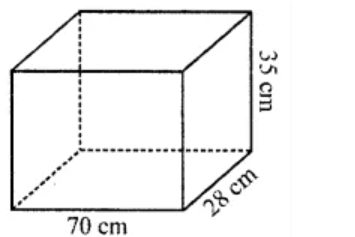# ML Aggarwal Solutions Class 8 Mathematics Solutions for Mensuration Exercise 18.4 in Chapter 18 - Mensuration

Find the total surface area of a solid cylinder of radius 5 cm and height 10 cm. Leave your answer in terms of n.

Given,

Radius of a solid cylinder (r) = 5 cm

Height (h) = 10 cm

Hence,

Total surface area = 2πrh + 2πr2

= 2rπ(h + r)

= 2π × 5(10 + 5)

= π × 10 × 15

= 150π cm2Related Questions

Lido

Courses

Teachers

Book a Demo with us

Syllabus

Maths
CBSE
Maths
ICSE
Science
CBSE

Science
ICSE
English
CBSE
English
ICSE
Coding

Terms & Policies

Selina Question Bank

Maths
Physics
Biology

Allied Question Bank

Chemistry
Connect with us on social media!